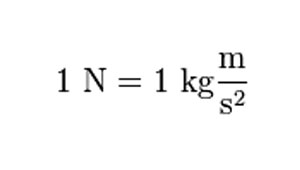# ieCivil

Engineering the Digital Civilization

## Saturday, 24 August 2019

Well most educated people know that Newton is the unit of force but rarely do we look into the practical aspects of the unit and its implication.

# Why are we discussing this?

It happened that one day, during a discussion with a colleague at the office somehow we started discussing how much is 1kN in Kg? Surprisingly, I was puzzled and couldn’t answer at the position. I have a habit of deriving stuff at the times when you need them, i.e. I tend to avoid remembering the constants and conversion factors which I could easily retrieve from a system or network. But, I understand that this could be very bad at certain instances and will include a few examples where it is absolutely recommended that you remember the values and stuff.

# Getting back to the topic

What is newton?
It is a unit of Force
What is kilonewton (kN)?
It is unit of force but here its’ multiplied 1000 times. The small “k” is a notation used to indicate the multiplier 1000.
Now let’s dig deeper & ask what is Force?
Force is that sensation or can be better defined as the interaction of different objects in the universe.
There are different kinds of forces such as
Gravity
Centrifugal Force
Magnetic Forces and the list continues…
Basically, we can define it as the mass of the object multiplied with acceleration.
If we consider Gravity, then the force will be Mass of the object multiplied by the acceleration due to gravity.
F = ma,
Here a = g (acceleration due to gravity)
Hence, F = mg
Where,
m = mass of the object in kg
g = acceleration due to gravity m/s2

If there is an object of mass 1 kg then it will exert a force of 1 x g ( 1 multiplied by g), on earth g is found to be approximately 9.81m/s2 ­and so the force will be 9.81 N
F = 1 kg X 9.81 m/s2
= 9.81 N
The unit newton N = kgm/s2 in SI units.
And so we can say that 1 kg force will be 9.81 N
N is the abbreviation used for to denote force.Image Credit: What is a Newton?

# Mass, Weight & Forces!

As a structural engineer, we usually consider a load of 2.5 kN/m2 for residential projects. How much is that weight actually? We as normal citizens are accustomed to seeing the weight of an object in kg. So lets’ try to visualize how much is that design consideration.Image Credits: Mass Vs Weight

Let’s differentiate between weight and mass:
1st Mass is the object’s inherent property that it has due to the molecules or atoms or element is it is composed of. This remains constant no matter what you do to it? Unless you do some quantum mechanical magic you won’t be able to change the mass of an object!
2nd Weight is the force that an object exerts due to its mass and this is commonly misinterpreted as the mass of the object. Weight and mass are often considered synonymous and that makes it rather difficult to understand when it is a force and when it is property.

Here’s a trick,
Generally, what we consider as the weight of an object, if it is measured using a scale of any kind then it is the mass of the object.

How?
When we measure reaction, it is a force!
When we balance the two sides it is mass!

Generally, the weighing scales that we use are calibrated such that when you stand and measure your weight it’ll show you your weight by dividing the force you exerted on the scale. In simple terms, if your weight is 100kg then the force you exerted on the scale will be 981 N, but the scale is calibrated in such a way that I’ll show you the mass by dividing it with 9.81 which is 100kg. So if you weigh yourself on a weighing scale that’s calibrated for the moon, then after returning back to earth and measuring yourself on a weighing scale calibrated for earth you’ll feel that you’ve actually gained a lot of weight. Here, mass is the quantity that is constant no matter where to take it, it balances out with an equal amount of the mass on the other side. So now you should know where you are getting your data from, if it’s from a scale you such probably find out if it is calibrated!

# Practicality

Our consideration of 2.5kN/m2, how much exactly is that in terms of mass/weight?
2.5kN/m2 = 2.5 x 1000 N/m2
1 kg = 9.81 N
1 N = 1/9.81 kg; Suppose 1/10 kg
2.5 kN/m2            = 2.5 X 1000 x 1/10 kg/m2
= 250 kg/m2

Assuming an adult man weighs around 65kg
Then 2.5 kN/m2 will be equivalent of 3.84 or 4 men standing in a box of 1 x 1 meter

Image Credit: Standing Crowd Density

Another way, a sack (bag) of rice grains is generally 20 – 25 kg then 2.5 kN/m2 will equate to 12.5 – 10 bags in a heap in an area of 1 meter by 1 meter.
Again all these values are near estimates.
Now floor finishes are generally considered to be 1kN/m2, how much does this equate in kg?
1kN/m2 = 1 x 1000 x 1/10 kg/m2     …according to the kg & N relationship that we established earlier
Then 1kN/m2 will be equivalent to 100 kg approximately.
This load will be as if you’re placing 2 bags of 50 kg cement on the area of 1 meter by 1 meter.

# Conclusions

It is essential that we relate the loading that we put on the structures with the actual life examples, while loadings are generally applied in the form of Newtons & kgf one should make an attempt to correlate the terms with physical/practical implications.